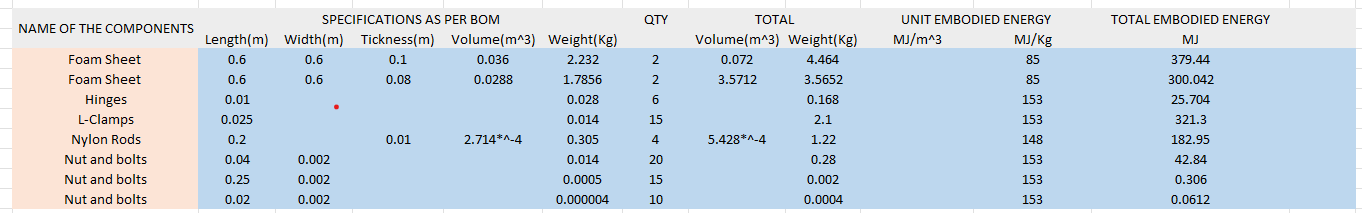# 8.SUSTANAIBILITY 2. - B-Division-2022-2023-Odd/Repo-11 GitHub Wiki

## 8.1 Embodied Energy Calculation.## 8.2 Embodied Energy Calculation(Alternate materials).

Calculations Volume = 0.6 x 0.6 x 0.01 = 0.036 m^3

Weight = Density x Volume = 62 x 0.036 = 2.232(kg)

Total Embodied Energy = Unit embodied Energy x Weight = 85 x 2.232 = 379.44 MJ